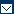## Calculating Gear Ratio or Debt/ Capital RatioTweet (function() { var po = document.createElement('script'); po.type = 'text/javascript'; po.async = true; po.src = 'https://apis.google.com/js/plusone.js'; var s = document.getElementsByTagName('script'); s.parentNode.insertBefore(po, s); })();

The gearing ratio or debt/Capital ratio, calculates the percentage of the total funds that represent the debt of the company. It is very important for best practice in financial management. It is also referred to as the debt-to-capital ratio, or simply – debt ratio. Calculating this ratio is a fundamental best practice that serves as a tool for analyzing how the company gets funded.

## Importance of the Gear Ratio

The figure obtained as the gear ratio is helpful in comparing the liabilities of the company in relation to the total capital. It provides companies the opportunity to review their dependence on debt financing that is usually external. In simpler terms, it defines the risks associated for stakeholders to be able to implement best practices for risk management.

Knowing the gear ratio also allows business owners to know their borrowing capacity. This is a recommended best practice that enables risk management to a great extent. This information also assists in best practices related to scheduling financial payments to settle the debts and leases. The debt/capital ratio is a best practice tool for analysts and bond rating companies to evaluate the creditworthiness of the company.

Assuming that the lowest value of gear ratio is the best achievement is a mistake and goes against risk management. This is what misleads most companies into taking larger loans to expand their business. Usually utilities that have higher capital requirements will have a higher gear ratio. Therefore, those companies that develop or manufacture new products or technology tend to have a higher gear ratio. This not a recommended best practice. It is important to mention that companies with higher debt must have positive earnings and a steady flow of cash to ensure risk management.

## Calculating the Gear Ratio

There are many approaches for calculating the gear ratio with best practices. However, the most common approach is to divide the sum of liabilities (or the long-term debts) by the sum total of assets. The sum total of assets is obtained as a sum of the liabilities and funds of the stockholders.

This is illustrated in the following mathematical equation:

Gear Ratio or debt/capital ratio = Sum of Liabilities ÷ Sum Total of Assets

## An Example of Calculation

Let us assume that the balance sheet of a company reports the total liabilities as \$10,000,000 and the total fund of stockholders as \$13,000,000. To ensure sufficient risk management it needs to know their gear ratio. The gear ratio or debt/capital ratio will be calculated as follows:

10,000,000 ÷ (10,000,000 + 13,000,000) = 10,000,000 ÷ (23,000,000) = 0.434. This can be expressed as a percentage by multiplying it by 100. Therefore the gear ratio will be reported as 43.4%.

Other approaches to calculate the gear ratio separate the different portion of the total of liabilities. However, for best practices this approach is not ideal because it becomes too complicated. Most experts consider those steps of the calculation as pointless. Some business people actually prefer to divide the total liabilities by the total funds.

Whichever approach one takes, the outcome is usually the same. However, ensuring that there are no errors is recommended and therefore sticking to simpler formulas is the ideal best practice.Contact Sitemap Links Copyright 2022 Best-Practice.com. All Rights Reserved.